# 320 Results

View
Selected filters:
• Algebra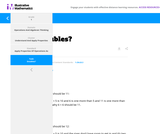Unrestricted Use
CC BY
Rating

This is a task from the Illustrative Mathematics website that is one part of a complete illustration of the standard to which it is aligned. Each task has at least one solution and some commentary that addresses important aspects of the task and its potential use.

Subject:
Algebra
Material Type:
Activity/Lab
Provider:
Illustrative Mathematics
Provider Set:
Illustrative Mathematics
Author:
Illustrative Mathematics
11/17/2020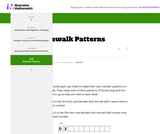Unrestricted Use
CC BY
Rating

This is a task from the Illustrative Mathematics website that is one part of a complete illustration of the standard to which it is aligned. Each task has at least one solution and some commentary that addresses important aspects of the task and its potential use.

Subject:
Algebra
Material Type:
Activity/Lab
Provider:
Illustrative Mathematics
Provider Set:
Illustrative Mathematics
Author:
Illustrative Mathematics
11/17/2020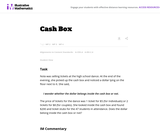Unrestricted Use
CC BY
Rating

The given solutions for this task involve the creation and solving of a system of two equations and two unknowns, with the caveat that the context of the problem implies that we are interested only in non-negative integer solutions. Indeed, in the first solution, we must also restrict our attention to the case that one of the variables is further even.

Subject:
Mathematics
Algebra
Material Type:
Activity/Lab
Provider:
Illustrative Mathematics
Provider Set:
Illustrative Mathematics
Author:
Illustrative Mathematics
01/18/2013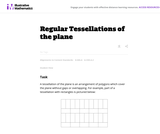Unrestricted Use
CC BY
Rating

This task examines the ways in which the plane can be covered by regular polygons in a very strict arrangement called a regular tessellation. These tessellations are studied here using algebra, which enters the picture via the formula for the measure of the interior angles of a regular polygon (which should therefore be introduced or reviewed before beginning the task). The goal of the task is to use algebra in order to understand which tessellations of the plane with regular polygons are possible.

Subject:
Mathematics
Algebra
Geometry
Material Type:
Activity/Lab
Provider:
Illustrative Mathematics
Provider Set:
Illustrative Mathematics
Author:
Illustrative Mathematics
01/21/2013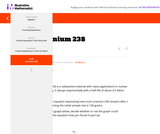Unrestricted Use
CC BY
Rating

This is a task from the Illustrative Mathematics website that is one part of a complete illustration of the standard to which it is aligned. Each task has at least one solution and some commentary that addresses important aspects of the task and its potential use.

Subject:
Algebra
Material Type:
Activity/Lab
Provider:
Illustrative Mathematics
Provider Set:
Illustrative Mathematics
Author:
Illustrative Mathematics
11/17/2020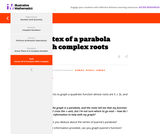Unrestricted Use
CC BY
Rating

This is a task from the Illustrative Mathematics website that is one part of a complete illustration of the standard to which it is aligned. Each task has at least one solution and some commentary that addresses important aspects of the task and its potential use.

Subject:
Algebra
Material Type:
Activity/Lab
Provider:
Illustrative Mathematics
Provider Set:
Illustrative Mathematics
Author:
Illustrative Mathematics
11/17/2020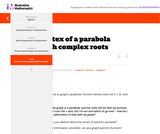Unrestricted Use
CC BY
Rating

This is a task from the Illustrative Mathematics website that is one part of a complete illustration of the standard to which it is aligned. Each task has at least one solution and some commentary that addresses important aspects of the task and its potential use.

Subject:
Algebra
Material Type:
Activity/Lab
Provider:
Illustrative Mathematics
Provider Set:
Illustrative Mathematics
Author:
Illustrative Mathematics
11/17/2020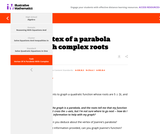Unrestricted Use
CC BY
Rating

This is a task from the Illustrative Mathematics website that is one part of a complete illustration of the standard to which it is aligned. Each task has at least one solution and some commentary that addresses important aspects of the task and its potential use.

Subject:
Algebra
Material Type:
Activity/Lab
Provider:
Illustrative Mathematics
Provider Set:
Illustrative Mathematics
Author:
Illustrative Mathematics
11/17/2020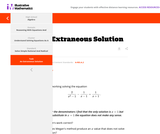Unrestricted Use
CC BY
Rating

This is a task from the Illustrative Mathematics website that is one part of a complete illustration of the standard to which it is aligned. Each task has at least one solution and some commentary that addresses important aspects of the task and its potential use.

Subject:
Algebra
Material Type:
Activity/Lab
Provider:
Illustrative Mathematics
Provider Set:
Illustrative Mathematics
Author:
Illustrative Mathematics
11/17/2020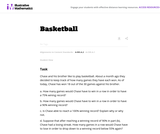Unrestricted Use
CC BY
Rating

This task provides a simple but interesting and realistic context in which students are led to set up a rational equation (and a rational inequality) in one variable, and then solve that equation/inequality for an unknown variable.

Subject:
Mathematics
Algebra
Material Type:
Activity/Lab
Provider:
Illustrative Mathematics
Provider Set:
Illustrative Mathematics
Author:
Illustrative Mathematics
12/15/2012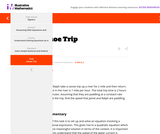Unrestricted Use
CC BY
Rating

This is a task from the Illustrative Mathematics website that is one part of a complete illustration of the standard to which it is aligned. Each task has at least one solution and some commentary that addresses important aspects of the task and its potential use.

Subject:
Algebra
Material Type:
Activity/Lab
Provider:
Illustrative Mathematics
Provider Set:
Illustrative Mathematics
Author:
Illustrative Mathematics
11/17/2020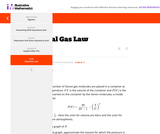Unrestricted Use
CC BY
Rating

This is a task from the Illustrative Mathematics website that is one part of a complete illustration of the standard to which it is aligned. Each task has at least one solution and some commentary that addresses important aspects of the task and its potential use.

Subject:
Algebra
Material Type:
Activity/Lab
Provider:
Illustrative Mathematics
Provider Set:
Illustrative Mathematics
Author:
Illustrative Mathematics
11/17/2020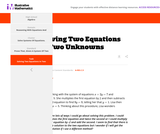Unrestricted Use
CC BY
Rating

This is a task from the Illustrative Mathematics website that is one part of a complete illustration of the standard to which it is aligned. Each task has at least one solution and some commentary that addresses important aspects of the task and its potential use.

Subject:
Algebra
Material Type:
Activity/Lab
Provider:
Illustrative Mathematics
Provider Set:
Illustrative Mathematics
Author:
Illustrative Mathematics
11/17/2020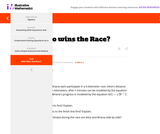Unrestricted Use
CC BY
Rating

This is a task from the Illustrative Mathematics website that is one part of a complete illustration of the standard to which it is aligned. Each task has at least one solution and some commentary that addresses important aspects of the task and its potential use.

Subject:
Algebra
Material Type:
Activity/Lab
Provider:
Illustrative Mathematics
Provider Set:
Illustrative Mathematics
Author:
Illustrative Mathematics
11/17/2020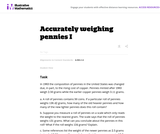Unrestricted Use
CC BY
Rating

This problem involves solving a system of algebraic equations from a context: depending how the problem is interpreted, there may be one equation or two.

Subject:
Mathematics
Algebra
Material Type:
Activity/Lab
Provider:
Illustrative Mathematics
Provider Set:
Illustrative Mathematics
Author:
Illustrative Mathematics
08/15/2012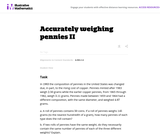Unrestricted Use
CC BY
Rating

This task is a somewhat more complicated version of "Accurately weighing pennies I'' as a third equation is needed in order to solve part (a) explicitly. Instead, students have to combine the algebraic techniques with some additional problem-solving (numerical reasoning, informed guess-and-check, etc.)

Subject:
Mathematics
Algebra
Material Type:
Activity/Lab
Provider:
Illustrative Mathematics
Provider Set:
Illustrative Mathematics
Author:
Illustrative Mathematics
08/15/2012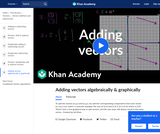Unrestricted Use
CC BY
Rating

To add the vectors (x₁,y₁) and (x₂,y₂), we add the corresponding components from each vector: (x₁+x₂,y₁+y₂). Here's a concrete example: the sum of (2,4) and (1,5) is (2+1,4+5), which is (3,9). There's also a nice graphical way to add vectors, and the two ways will always result in the same vector.​​

Subject:
Algebra
Material Type:
Lesson
Provider:
Provider Set:
Author:
Salman Khan
11/17/2020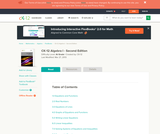Conditional Remix & Share Permitted
CC BY-NC-SA
Rating

A work in progress, CK-12's Algebra I Second Edition is a clear presentation of algebra for the high school student. Topics include: Equations and Functions, Real Numbers, Equations of Lines, Solving Systems of Equations and Quadratic Equations.

Subject:
Algebra
Functions
Material Type:
Textbook
Provider:
CK-12 Foundation
Provider Set:
CK-12 FlexBook
Author:
Andrew
Annamaria
Anne
Eve
Farbizio
Gloag
Rawley
12/03/2010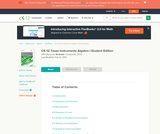Conditional Remix & Share Permitted
CC BY-NC-SA
Rating

CK-12's Texas Instruments Algebra I Student Edition Flexbook allows students to better utilize a graphing calculator in understanding the fundamental concepts of algebra.

Subject:
Algebra
Material Type:
Textbook
Provider:
CK-12 Foundation
Provider Set:
CK-12 FlexBook
Author:
Jordan, Lori
12/08/2010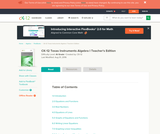Conditional Remix & Share Permitted
CC BY-NC-SA
Rating

CK-12's Texas Instruments Algebra I Teacher's Edition Flexbook allows an Instructor to teach students to better utilize a graphing calculator in understanding the fundamental concepts of algebra.

Subject:
Algebra
Material Type:
Textbook
Provider:
CK-12 Foundation
Provider Set:
CK-12 FlexBook
Author:
Jordan, Lori
12/13/2010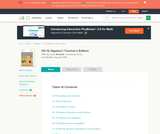Conditional Remix & Share Permitted
CC BY-NC-SA
Rating

CK-12 Foundation's Algebra I Teacher's Edition FlexBook complements CK-12's Algebra I Student Edition. The solution and assessment guides are available upon request.

Subject:
Algebra
Material Type:
Textbook
Provider:
CK-12 Foundation
Provider Set:
CK-12 FlexBook
Author:
Fay-Zenk, Mary
McFarland, Andrew
11/06/2009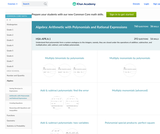Conditional Remix & Share Permitted
CC BY-NC-SA
Rating

This site teaches Arithmetic with Polynomials and Rational Expressions to High Schoolers through a series of 4333 questions and interactive activities aligned to 26 Common Core mathematics skills.

Subject:
Mathematics
Algebra
Material Type:
Activity/Lab
Interactive
Provider:
Provider Set:
11/17/2020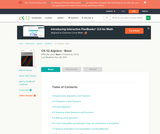Conditional Remix & Share Permitted
CC BY-NC-SA
Rating

CK-12 Foundation's Basic Algebra FlexBook is an introduction to the algebraic topics of functions, equations, and graphs for middle-school and high-school students.

Subject:
Algebra
Functions
Material Type:
Textbook
Provider:
CK-12 Foundation
Provider Set:
CK-12 FlexBook
Author:
Farbizio, Annamaria
Gloag, Andrew
Gloag, Anne
Kramer, Melissa
09/21/2010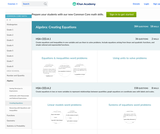Conditional Remix & Share Permitted
CC BY-NC-SA
Rating

This site teaches High Schoolers how to create equations through a series of 298 questions and interactive activities aligned to 5 Common Core mathematics skills.

Subject:
Mathematics
Algebra
Material Type:
Activity/Lab
Interactive
Provider:
Provider Set:
11/17/2020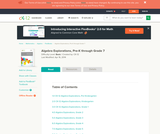Unrestricted Use
CC BY
Rating

CK-12 Algebra Explorations is a hands-on series of activities that guides students from Pre-K to Grade 7 through algebraic concepts.

Subject:
Algebra
Functions
Material Type:
Activity/Lab
Lesson Plan
Textbook
Provider:
CK-12 Foundation
Provider Set:
CK-12 FlexBook
Author:
Mary Cavanagh, Carol Findell, Carole Greenes
10/04/2011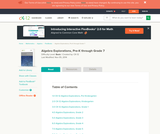Conditional Remix & Share Permitted
CC BY-NC-SA
Rating

CK-12 Algebra Explorations is a hands-on series of activities that guides students from Pre-K to Grade 7 through algebraic concepts.

Subject:
Algebra
Material Type:
Activity/Lab
Teaching/Learning Strategy
Provider:
CK-12 Foundation
Provider Set:
CK-12 FlexBook
Author:
Carol ­ Findell, Mary ­Cavanagh, Carole ­Greenes
02/23/2012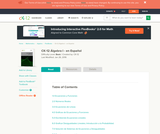Conditional Remix & Share Permitted
CC BY-NC-SA
Rating

CK-12 Foundation's Algebra FlexBook is an introduction to algebraic concepts for the high school student. Topics include: Equations & Functions, Real Numbers, Equations of Lines, Solving Systems of Equations & Quadratic Equations.

Subject:
Algebra
Material Type:
Textbook
Provider:
CK-12 Foundation
Provider Set:
CK-12 FlexBook
Author:
Gloag, Andrew
Gloag, Anne
11/23/2010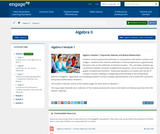Conditional Remix & Share Permitted
CC BY-NC-SA
Rating

Students connect polynomial arithmetic to computations with whole numbers and integers.  Students learn that the arithmetic of rational expressions is governed by the same rules as the arithmetic of rational numbers.  This unit helps students see connections between solutions to polynomial equations, zeros of polynomials, and graphs of polynomial functions.  Polynomial equations are solved over the set of complex numbers, leading to a beginning understanding of the fundamental theorem of algebra.  Application and modeling problems connect multiple representations and include both real world and purely mathematical situations.

Subject:
Algebra
Material Type:
Module
Provider:
New York State Education Department
Provider Set:
EngageNY
05/14/2013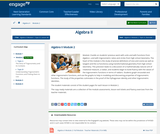Conditional Remix & Share Permitted
CC BY-NC-SA
Rating

Module 2 builds on students previous work with units and with functions from Algebra I, and with trigonometric ratios and circles from high school Geometry. The heart of the module is the study of precise definitions of sine and cosine (as well as tangent and the co-functions) using transformational geometry from high school Geometry. This precision leads to a discussion of a mathematically natural unit of rotational measure, a radian, and students begin to build fluency with the values of the trigonometric functions in terms of radians. Students graph sinusoidal and other trigonometric functions, and use the graphs to help in modeling and discovering properties of trigonometric functions. The study of the properties culminates in the proof of the Pythagorean identity and other trigonometric identities.

Subject:
Algebra
Material Type:
Module
Provider:
New York State Education Department
Provider Set:
EngageNY
08/15/2014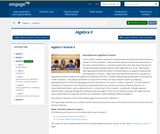Conditional Remix & Share Permitted
CC BY-NC-SA
Rating

In this module, students synthesize and generalize what they have learned about a variety of function families.  They extend the domain of exponential functions to the entire real line (N-RN.A.1) and then extend their work with these functions to include solving exponential equations with logarithms (F-LE.A.4).  They explore (with appropriate tools) the effects of transformations on graphs of exponential and logarithmic functions.  They notice that the transformations on a graph of a logarithmic function relate to the logarithmic properties (F-BF.B.3).  Students identify appropriate types of functions to model a situation.  They adjust parameters to improve the model, and they compare models by analyzing appropriateness of fit and making judgments about the domain over which a model is a good fit.  The description of modeling as, the process of choosing and using mathematics and statistics to analyze empirical situations, to understand them better, and to make decisions, is at the heart of this module.  In particular, through repeated opportunities in working through the modeling cycle (see page 61 of the CCLS), students acquire the insight that the same mathematical or statistical structure can sometimes model seemingly different situations.

Subject:
Algebra
Material Type:
Module
Provider:
New York State Education Department
Provider Set:
EngageNY
09/16/2014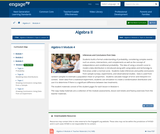Conditional Remix & Share Permitted
CC BY-NC-SA
Rating

Students build a formal understanding of probability, considering complex events such as unions, intersections, and complements as well as the concept of independence and conditional probability.  The idea of using a smooth curve to model a data distribution is introduced along with using tables and techonolgy to find areas under a normal curve.  Students make inferences and justify conclusions from sample surveys, experiments, and observational studies.  Data is used from random samples to estimate a population mean or proportion.  Students calculate margin of error and interpret it in context.  Given data from a statistical experiment, students use simulation to create a randomization distribution and use it to determine if there is a significant difference between two treatments.

Subject:
Algebra
Material Type:
Module
Provider:
New York State Education Department
Provider Set:
EngageNY
03/24/2016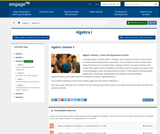Conditional Remix & Share Permitted
CC BY-NC-SA
Rating

In earlier grades, students define, evaluate, and compare functions and use them to model relationships between quantities. In this module, students extend their study of functions to include function notation and the concepts of domain and range. They explore many examples of functions and their graphs, focusing on the contrast between linear and exponential functions. They interpret functions given graphically, numerically, symbolically, and verbally; translate between representations; and understand the limitations of various representations.

Subject:
Algebra
Material Type:
Module
Provider:
New York State Education Department
Provider Set:
EngageNY
09/17/2013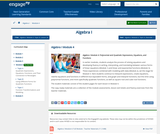Conditional Remix & Share Permitted
CC BY-NC-SA
Rating

In earlier modules, students analyze the process of solving equations and developing fluency in writing, interpreting, and translating between various forms of linear equations (Module 1) and linear and exponential functions (Module 3). These experiences combined with modeling with data (Module 2), set the stage for Module 4. Here students continue to interpret expressions, create equations, rewrite equations and functions in different but equivalent forms, and graph and interpret functions, but this time using polynomial functions, and more specifically quadratic functions, as well as square root and cube root functions.

Subject:
Algebra
Material Type:
Module
Provider:
New York State Education Department
Provider Set:
EngageNY
09/17/2013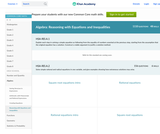Conditional Remix & Share Permitted
CC BY-NC-SA
Rating

This site teaches Reasoning with Equations and Inequalities to High Schoolers through a series of 5909 questions and interactive activities aligned to 36 Common Core mathematics skills.

Subject:
Mathematics
Algebra
Material Type:
Activity/Lab
Interactive
Provider:
Provider Set:
11/17/2020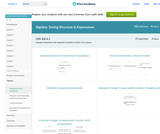Conditional Remix & Share Permitted
CC BY-NC-SA
Rating

This site teaches Structure in Algebraic Expressions to High Schoolers through a series of 3482 questions and interactive activities aligned to 26 Common Core mathematics skills.

Subject:
Mathematics
Algebra
Material Type:
Activity/Lab
Interactive
Provider:
Provider Set:
11/17/2020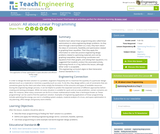Educational Use
Rating

Students learn about linear programming (also called linear optimization) to solve engineering design problems. As they work through a word problem as a class, they learn about the ideas of constraints, feasibility and optimization related to graphing linear equalities. Then they apply this information to solve two practice engineering design problems related to optimizing materials and cost by graphing inequalities, determining coordinates and equations from their graphs, and solving their equations. It is suggested that students conduct the associated activity, Optimizing Pencils in a Tray, before this lesson, although either order is acceptable.

Subject:
Mathematics
Algebra
Geometry
Material Type:
Lesson
Provider:
TeachEngineering
Provider Set:
TeachEngineering
Author:
Andi Vicksman
Malinda Zarske
Nathan Coyle
Russell Anderson
Ryan Sullivan
02/17/2021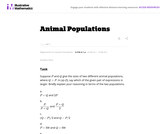Unrestricted Use
CC BY
Rating

In this task students have to interpret expressions involving two variables in the context of a real world situation. All given expressions can be interpreted as quantities that one might study when looking at two animal populations.

Subject:
Mathematics
Algebra
Material Type:
Activity/Lab
Provider:
Illustrative Mathematics
Provider Set:
Illustrative Mathematics
Author:
Illustrative Mathematics
05/01/2012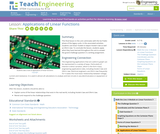Educational Use
Rating

This final lesson in the unit culminates with the Go Public phase of the legacy cycle. In the associated activities, students use linear models to depict Hooke's law as well as Ohm's law. To conclude the lesson, students apply they have learned throughout the unit to answer the grand challenge question in a writing assignment.

Subject:
Mathematics
Algebra
Engineering
Material Type:
Lesson Plan
Provider:
TeachEngineering
Provider Set:
TeachEngineering
Author:
Aubrey McKelvey
09/18/2014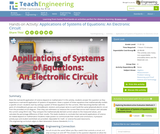Educational Use
Rating

Does the real-world application of science depend on mathematics? In this activity, students answer this question as they experience a real-world application of systems of equations. Given a system of linear equations that mathematically models a specific circuit—students start by solving a system of three equations for the currents. After becoming familiar with the parts of a breadboard, groups use a breadboard, resistors and jumper wires to each build the same (physical) electric circuit from the provided circuit diagram. Then they use voltmeters to measure the current flow across each resistor and calculate the current using Ohm’s law. They compare the mathematically derived current values to the measured values, and calculate the percentage difference of their results. This leads students to conclude that real-world applications of science do indeed depend on mathematics! Students make posters to communicate their results and conclusions. A pre/post-activity quiz and student worksheet are provided. Adjustable for math- or science-focused classrooms.

Subject:
Algebra
Measurement and Data
Engineering
Material Type:
Activity/Lab
Provider:
TeachEngineering
Provider Set:
Activities
Author:
Marianne Livezey
11/01/2017Educational Use
Rating

The CyberSquad tries to figure out a table arrangement for 20 workers in this video from Cyberchase.

Subject:
Mathematics
Algebra
Material Type:
Lecture
Provider:
PBS LearningMedia
Author:
U.S. Department of Education
WNET